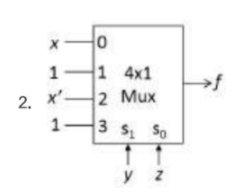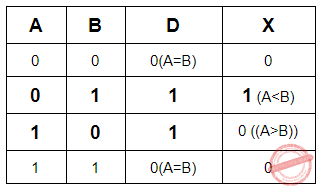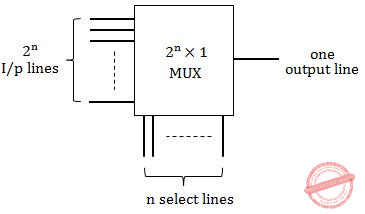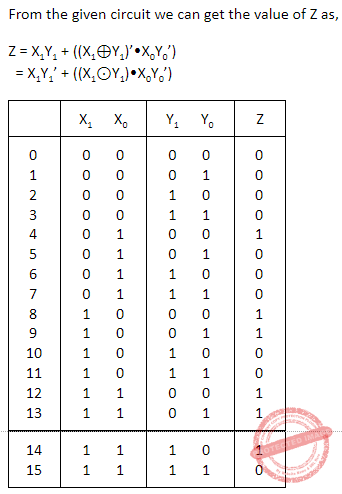## Combinational-Circuit

 Question 1

A multiplexer is placed between a group of 32 registers and an accumulator to regulate data movement such that at any given point in time the content of only one register will move to the accumulator. The minimum number of select lines needed for the multiplexer is _____.

 A 5
Digital-Logic-Design       Combinational-Circuit       GATE 2020
Question 1 Explanation:
Number of registers is 32. Only one register has to be selected at any instant of time.
A 25x1 Multiplexer with 5 select lines selects one of the 32(= 25) registers at a time depending on the selection input.
The content from the selected register will be transferred through the output line to the Accumulator.
 Question 2

If there are m input lines and n output lines for a decoder that is used to uniquely address a byte addressable 1 KB RAM, then the minimum value of m + n is ____.

 A 1034
Digital-Logic-Design       Combinational-Circuit       GATE 2020
Question 2 Explanation:
The size of the decoder required is 10 x 210 i.e., 10 x 1024.
Each output line of the decoder is connected to one of the 1K(= 1024) rows of RAM.
Each row stores 1 Byte.
m=10 and n=1024
 Question 3
Which one of the following circuits implements the Boolean function given below?ABCDDigital-Logic-Design       Combinational-Circuit       GATE 2021 CS-Set-2
Question 3 Explanation:Question 4
Consider a digital display system (DDS) shown in the figure that displays the contents of register X. A 16-bit code word is used to load a word in X, either from S or from R. S is a 1024-word memory segment and R is a 32-word register file. Based on the value of mode bit M, T selects an input word to load in X. P and Q interface with the corresponding bits in the code word to choose the addressed word. Which one of the following represents the functionality of P, Q, and T?A P is 10:1 multiplexer; Q is 5:1 multiplexer; T is 2:1 multiplexer B P is 10:2 ^10 decoder; Q is 5:2^ 5 decoder; T is 2:1 encoder C P is 10:2^ 10 decoder; Q is 5:2^ 5 decoder; T is 2:1 multiplexer D P is 1:10 de-multiplexer; Q is 1:5 de-multiplexer; T is 2:1 multiplexer
Digital-Logic-Design       Combinational-Circuit       GATE 2022
Question 4 Explanation:
P is a 10:2^10 decoder that takes a 10-bit address from S-address as input and enable one of the 1024 words of the S memory.
Q is a 5:2^5 decoder that takes a 5-bit address from R-address as input and enable one of the 32 words of the R memory.
T is a 2x1 Multiplexer that select one of the 2 inputs and transmit it as output.
 Question 5
The circuit shown in the given figure is aA full adder B full subtractor C shift register D decade counter
Digital-Logic-Design       Combinational-Circuit       ISRO-2007
Question 5 Explanation:
The above diagram is full subtractor. The equation is D=X⊕Y⊕Bin and Bout=X'Bin+X'Y+YBin
 Question 6
By using an eight-bit optical encoder the degree of resolution that can be obtained is (approximately)
 A 1.8o B 3.4o C 2.8o D 1.4o
Digital-Logic-Design       Combinational-Circuit       ISRO-2007
Question 6 Explanation:
An optical encoder is an electromechanical device which has an electrical output in digital form proportional to the angular position of the input shaft.
Optical encoders enable an angular displacement to be converted directly into a digital form.
Encoder resolution is often referred to in bits, which are binary units: a 16 bit resolution rotary encoder will have 65,536 (216) increments per turn, or PPR.
In the given question, 8-bit optical encoder will have 28 increments Resolution = 360/2n = 360/28 = 1.4o
 Question 7
For a binary half-subtractor having two inputs A and B, the correct set of logical expressions for the outputs D (= A minus B) and X (=borrow) are
 A D = AB + A’B , X = A’B B D = A’B + AB’ , X = AB’ C D = A’B + AB’ , X = A’B D D = AB + A’B , X = AB’
Digital-Logic-Design       Combinational-Circuit       ISRO-2016
Question 7 Explanation:
The function table for the Half Subtractor is as followsA-B= D= A’B + AB’
X= A’B
 Question 8
Suppose you want to build a memory with 4-byte words with a capacity of 221 bits.
What is the type of decoder required if the memory is built using 2K x 8 RAM chips?
 A 5 to 32 B 6 to 64 C 4 to 64 D 7 to 128
Digital-Logic-Design       Combinational-Circuit       ISRO CS 2014
Question 8 Explanation:
In digital electronics, a binary decoder is a combinational logic circuit that converts binary information from the n coded inputs to a maximum of 2n unique outputs.
We need to built memory with 4 byte words with a capacity of 221bits
So, number of 4-byte words memory = total number of bits / number of byte words
= 221/(4*bytes)
= 221/(4*8)
=221/32=221/25
= 216 words
Given RAM chips are of size = 2K x 8
Memory to be built using these RAM chips = 216
Required RAM chips = (216 x 32) / (2K x 8) = 32 x 4
So, RAM chips contains 32 rows and each row with 4 columns.
A Decoder is required to select a specific row and multiplexer is required to select a particular column. 5 to 32 Decoder will be required to select the desired row.
 Question 9

Match the terms in List-I with the options given in List-II :

```    List-I                      List-II
(a) Decoder             (i) 1 line to 2n lines
(b) Multiplexer        (ii) n lines to 2n lines
(c) De multiplexer    (iii) 2n lines to 1 line
(iv) 2n lines to 2n-1 lines

```
 A (a)-(ii), (b)-(i), (c)-(iii) B (a)-(ii), (b)-(iii), (c)-(i) C (a)-(ii), (b)-(i), (c)-(iv) D (a)-(iv), (b)-(ii), (c)-(i)
Digital-Logic-Design       Combinational-Circuit       UGC-NET CS 2018 JUNE Paper-2
Question 9 Explanation:
Decoder:Multiplexer: Many to OneDemultiplexer: One to ManyQuestion 10
Minimum number of 2x1 multiplexers required to realize the following function,
f = A’B’C + A’B’C’
Assume that inputs are available only in true form and Boolean constant 1 and 0 are available.
 A 1 B 2 C 3 D 7
Digital-Logic-Design       Combinational-Circuit       ISRO CS 2015
Question 10 Explanation:
Given function f = A’B’C + A’B’C’
=A’B’(C+C’) (C+C’=1)
=A’B’
=(A+B)’
So we can implement with minimum Two number of 2x1 multiplexers
 Question 11
A device which converts BCD to seven segment is called____
 A Encoder B Decoder C Decoder D Demultiplexer
Digital-Logic-Design       Combinational-Circuit       KVS 22-12-2018 Part-B
Question 11 Explanation:
A Display Decoder is a combinational circuit which decodes and n-bit input value into a number of output lines to drive a display
 Question 12
A half-adder is also known as :
 A AND Circuit B NAND Circuit C NOR Circuit D EX-OR Circuit
Digital-Logic-Design       Combinational-Circuit       UGC NET CS 2005 Dec-Paper-2
Question 12 Explanation:
→ The half adder adds two single binary digits A and B. It has two outputs, sum (S) and carry (C). The carry signal represents an overflow into the next digit of a multi-digit addition. The value of the sum is 2C + S. The simplest half-adder design incorporates an XOR gate for S and an AND gate for C. The Boolean logic for the sum (in this case S) will be A′B + AB′ whereas for the carry (C) will be AB.Question 13
Which of the following expression removes static hazard from a two level AND - OR gate implementation of xy +zx’
 A xy +zx’ B xy + zx’+ wxy C xy +zx’ +yz D xy + zx’ +wz
Digital-Logic-Design       Combinational-Circuit       UGC NET CS 2006 June-Paper-2
Question 13 Explanation:
→ A static hazard occurs if a circuit produces incorrect output value momentarily before stabilizing to its correct value.
→ Generally Hazard occurs due to different delays in different paths of the circuit.
→ In the expression xy +zx’ the variable x is in true form in one term(xy) and in complement in other term(zx').
→ Delay occurs due to the presence of NOT gate. If input xyz=111 then output is 1. If input xyz=011 then output stays momentarily in state 0 then settles in state 1.
→ Adding the term yz(select y from xy and z from zx') eliminates the hazard.
 Question 14
Which of the following statements are true ?
I. A circuit that adds two bits, producing a sum bit and a carry bit is called half adder.
II. A circuit that adds two bits, producing a sum bit and a carry bit is called full adder.
III. A circuit that adds two bits and a carry bit producing a sum bit and a carry bit is called full adder.
IV. A device that accepts the value of a Boolean variable as input and produces its complement is called an inverter.
 A I & II B II & III C I, II, III D I, III & IV
Digital-Logic-Design       Combinational-Circuit       UGC NET CS 2013 Dec-paper-2
Question 14 Explanation:
TRUE: A circuit that adds two bits, producing a sum bit and a carry bit is called half adder.
FALSE: A circuit that adds two bits, producing a sum bit and a carry bit is called full adder.
It is false because it is half adder
TRUE: A circuit that adds two bits and a carry bit producing a sum bit and a carry bit is called full adder.
TRUE: A device that accepts the value of a Boolean variable as input and produces its complement is called an inverter.
 Question 15
A multiplexer is a logic circuit that
 A accepts one input and gives several output B accepts many inputs and gives many output C accepts many inputs and gives one output D accepts one input and gives one output
Digital-Logic-Design       Combinational-Circuit       UGC NET CS 2011 June-Paper-2
Question 15 Explanation:
A multiplexer is a logic circuit that accepts many inputs and gives one output. Demultiplex is just reverse of it.
 Question 16
In order to implement a n variable switching function, a MUX must have :
 A 2n inputs B 2n+1 inputs C 2n-1 inputs D 2n-1 inputs
Digital-Logic-Design       Combinational-Circuit       UGC NET CS 2009-June-Paper-2
Question 16 Explanation:
In order to implement a n variable switching function, a MUX must have 2n inputs.
 Question 17
If four 4 input multiplexers drive a 4 input multiplexer, we get a :
 A 16 input MUX B 8 input MUX C 4 input MUX D 2 input MUX
Digital-Logic-Design       Combinational-Circuit       UGC NET CS 2008 Dec-Paper-2
Question 17 Explanation:
If ‘n’ input lines, it will generate 2n output lines.
Given, n=4
= 2n output lines.
= 24
= 16 output lines.
 Question 18
The output of the following combinational circuit.A X.Y B X+Y C X⊕Y D (X⊕Y)’
Digital-Logic-Design       Combinational-Circuit       UGC NET CS 2016 Aug- paper-3
Question 18 Explanation:Question 19
The three outputs x1x2x3 from the 8 X 3 priority encoder are used to provide a vector address of the form 101x1x2x300. What is the second highest priority vector address in hexadecimal if the vector addresses are starting from the one with the highest priority ?
 A BC B A4 C BD D AC
Digital-Logic-Design       Combinational-Circuit       UGC NET CS 2015 Dec - paper-3
Question 19 Explanation:Question 20
How many address lines and data lines are required to provide a memory capacity of 16K x 16?
 A 10,4 B 16,16 C 14,16 D 4,16
Digital-Logic-Design       Combinational-Circuit       UGC NET June-2019 CS Paper-2
Question 20 Explanation:
16K x 16 means we have 2​ 14​ address lines and 16 data lines.
In order to provide ​ 2 14 address lines we need 14 address lines and in order to provide 16 data lines we need 16 data lines.
 Question 21
The parallel bus arbitration technique uses an external priority encoder and a decoder. Suppose, a parallel arbiter has 5 bus arbiters. What will be the size of priority encoder and decoder respectively?
 A 4x2, 2x4 B 2x4, 4x2 C 3x8, 8x3 D 8x3, 3x8
Digital-Logic-Design       Combinational-Circuit       UGC NET June-2019 CS Paper-2
Question 21 Explanation:Question 22
Which of the following represents the function of a Multiplexer?
 A Y= A+ B B Y = A | B C Y = A & B D Y = S ? A : B
Digital-Logic-Design       Combinational-Circuit       CIL Part - B
Question 22 Explanation:
Multiplexer : It also known as a data selector, is a device that selects between several input signals and forwards it to a single output line. A multiplexer of 2n inputs has n select lines, which are used to select which input line to send to the output. Here in fourth option “S” is used as select line to select either “A” or “B”.
A multiplexer can be used to implement if-else statements.
 Question 23
Consider a memory unit of size 96k x 16, where first component represents the total number of words and that the second component represents the number of bits per word. What will be the number if address lines and input-output data lines?
 A 16 address lines, 7 data lines B 7 address lines, 16 data lines C 17 address lines, 16 data lines D 16 address lines, 17 data lines
Digital-Logic-Design       Combinational-Circuit       CIL Part - B
Question 23 Explanation:
Given memory unit is 96K x 16
96 is greater than 64 and less than 128 ,So we need to consider 128.
96= 2x2x2x2x2x3 which is equivalent to 2 ^ 7
96K which is 2^7 x 2^10 =2^17
So address lines are 17
Word size is 16
So data lines are 2 ^16.
So option C is correct .
 Question 24
The following circuit compares two 2-bit binary numbers, X and Y represented by X1X0 and Y1Y0 respectively. ( X0 and Y0 represent Least Significant Bids)Under what condition Z will be 1?
 A X > Y B X < Y C X = Y D X! = Y
Digital-Logic-Design       Combinational-Circuit       ISRO CS 2020       Video-Explanation
Question 24 Explanation:
Output of OR gate is 1 if at least one of the two inputs is1.
Case 1: X1=1 and Y1=0 which implies X > Y.
or Case 2: X1=Y1 and (X0=1 and Y0=0) which implies X > Y.
Z=1 in both of the above cases which implies X > Y.
 Question 25
In a 8-bit ripple carry adder using identical full adders, each full adder takes 34ns for computing sum. If the time taken for 8-bit addition is 90ns, find the time taken by each full adDer to find carry.
 A 6 ns B 7 ns C 10 ns D 8 ns
Digital-Logic-Design       Combinational-Circuit       ISRO CS 2020       Video-Explanation
Question 25 Explanation:
Consider n-bit Ripple Carry Adder.
Total Delay = Delay_Sum + (n-1) Delay_Carry
Here, n=8.
90ns = 34 ns + 7 * Delay_Carry
56ns = 7 * Delay_Carry
Delay_Carry= 8ns
 Question 26
Following Multiplexer circuits is equivalent toA Sum equation of full adder B Carry equation of full adder C Borrow equation for full subtraction D Difference equation of a full subtractor E Both A and D
Digital-Logic-Design       Combinational-Circuit       ISRO CS 2020       Video-Explanation
Question 26 Explanation:
The output Y of the given MUX is the equation for the difference of a full subtractor and Sum of a Full Adder.
Y is the difference between A and B where C is the Barrow.
Y is the Sum of A and B where C is the Carry_in.Y = B’A’C + B’AC’ + BA’C’ + BAC
Y = (A ⊕ B ⊕ C)
 Question 27
Consider the following circuitThe function by the network above is
 A (AB)’E + EF + (CD)’F B (E’ + ABF’)(C+D+F’) C ((AB)’+E)(E’+F’)(C+D+F’) D (A+B)E’ + (EF)’ + CDF’
Digital-Logic-Design       Combinational-Circuit       ISRO CS 2020       Video-Explanation
Question 27 Explanation:
[ ((AB)’ E) + (EF) + (F(C+D)’) ]’
= ((AB)’ E)’ (EF)’ (F(C+D)’)’
= (AB+E’)(E’+F’)(F’+C+D)
= (ABE’ + ABF’ + E’ + E’F’)(F’+C+D)
= (ABF’+ E’(AB+1+F’))(F’+C+D)
= (ABF’+E’) (F’+C+D)
 Question 28
The number of full and half adders required to add 16 – bit number is
 A 8 half addres, 8 full adders B 1 half adder, 15 full adders C 16 half adders, 0 full adders D 4 half adders, 12 full-adders
Digital-Logic-Design       Combinational-Circuit       APPSC-2016-DL-CS
Question 28 Explanation:Question 29
Consider the implementation of a Boolean function of ‘n’ variables using only one multiplexer and one inverter. The minimum specification of size of multiplexer required is ____________
 A 2n –input & I -output B 1 –output & 2n output C 2n-1 input & 1 output D 2n+1 input & 1 output
Digital-Logic-Design       Combinational-Circuit       APPSC-2016-DL-CS
Question 29 Explanation:
The minimum specification of size of multiplexer required is 2^(n-1) input & 1 output in which n-1 variables will be given to n-1 select lines and remaining one variable will be given to input lines.
 Question 30

The number of full and half adders required to add 16-bit numbers are

 A 8 half adders, 8 full adders B 1 half adder, 15 full adders C 16 half adders, 0 full adders D half adders, 12 full adders
Digital-Logic-Design       Combinational-Circuit       APPSC-2016-DL-CA
Question 30 Explanation:
For the first 15 bits we need 15 full adders and for LSB we need 1 half adder.
 Question 31

The number of control lines for a 8 to 1 multiplexer is

 A 2 B 3 C 4 D 5
Digital-Logic-Design       Combinational-Circuit       APPSC-2016-DL-CA
Question 31 Explanation:
No. of control lines for 8 to 1 multiplexer is log2 8 = 3.
 Question 32

The gates required to build a half adder are

 A Ex-OR gate and NOR gate B Ex-OR gate and OR gate C Ex-OR gate and AND gate D Four NAND gates
Digital-Logic-Design       Combinational-Circuit       APPSC-2016-DL-CA
Question 32 Explanation:
In half adder for sum value we need 1 XOR gate and for carry value we need 1 AND gate.
 Question 33

In order to implement an n-variable switching function, a MUX must have

 A 2n inputs B 2n + inputs C 2n-1 inputs D 2n – 1 inputs
Digital-Logic-Design       Combinational-Circuit       APPSC-2016-DL-CA
Question 33 Explanation:
In order to implement an n-variable switching function, a MUX must have 2n-1 inputs, where we can give n-1 variables and remaining 1 we can give to the select lines.
 Question 34
A terminal multiplexer has six 1200 bps terminals and ‘n’ 300 bps terminals connected to it. If the outgoing line is 9600 bps, what is the value of n ?
 A 4 B 8 C 16 D 28
Digital-Logic-Design       Combinational-Circuit       UGC NET CS 2014 June-paper-3
Question 34 Explanation:
According to given data,
(6*1200)+(n*300)= 9600
7200+300n = 9600
300n = 9600-7200
300n= 2400
n= 8
 Question 35
The logic circuit given below is used to compare two unsigned 2-bit numbers, X1X0 = X and Y1 Y0 = Y, where Xo and Yo are the least significant bits. (A small circle on any line in a logic diagram indicates logical NOT). Which of the following always makes the output Z have the value 1?A X >Y B X < Y C X =Y D X ≥ Y
Digital-Logic-Design       Combinational-Circuit       HCU PHD CS MAY 2019
Question 35 Explanation:So from the above table we can clearly see that, for X>Y the value of Z is 1.
 Question 36A a B b C c D d E None of the above
Digital-Logic-Design       Combinational-Circuit       TIFR PHD CS & SS 2020
 Question 37
A clamp gate is an analog gate parametrized by two real numbers a and b, and denoted as clampa,b. It takes as input two non-negative real numbers x and y. Its output is defined asConsider circuits composed only of clamp gates, possibly parametrized by different pairs (a, b) of real numbers. How many clamp gates are needed to construct a circuit that on input non-negative reals x and y outputs the maximum of x and y?
 A 1 B 2 C 3 D 4 E No circuit composed only of clamp gates can compute the max function.
Digital-Logic-Design       Combinational-Circuit       TIFR PHD CS & SS 2020
 Question 38
Encoders are made by three ______ gates.
 A AND B OR C NAND D XOR
Digital-Logic-Design       Combinational-Circuit       NIC-NIELIT STA 2020
Question 38 Explanation:
Encoders are made by three OR gates
Encoder may also be designed by using 3 NAND gates. Thus both option B,C are correct.
There are 38 questions to complete.